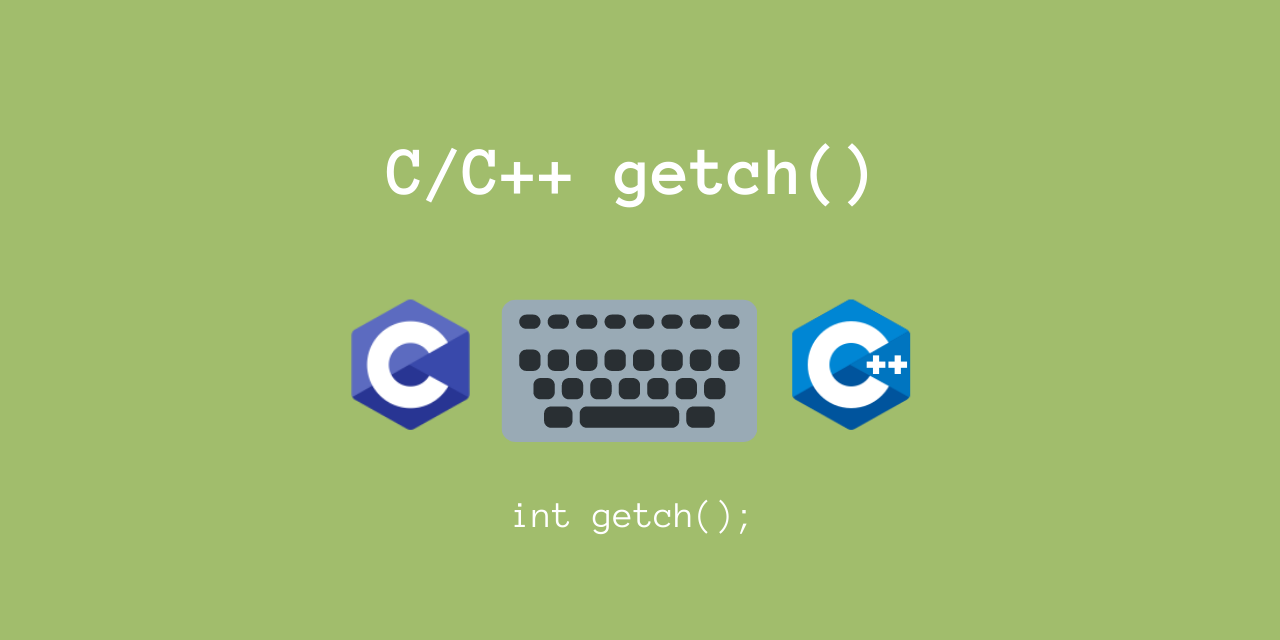# Using the getch() function in C/C++

Published on August 3, 2022By Vijaykrishna Ram
Developer and author at DigitalOcean.While we believe that this content benefits our community, we have not yet thoroughly reviewed it. If you have any suggestions for improvements, please let us know by clicking the “report an issue“ button at the bottom of the tutorial.

In this article, we’ll take a look at using the getch() function in C/C++.

The getch() function is very useful if you want to read a character input from the keyboard.

While this is not a part of the C standard, this is still a POSIX C function. So, we can still use this function from Windows / Linux / Mac.

Let’s take a look at using this function, using a few examples.

## Basic Syntax of getch() in C/C++

This function takes in a single character from the standard input (`stdin`), and returns an integer.

This is there as part of the `<conio.h>` header file, so you must include it in your program.

``````#include <conio.h>
int getch();
``````

This function does not take any parameters.

Here, `getch()` returns the ASCII value of the character read from `stdin`.

For example, if we give the character ‘0’ as input, it will return the ASCII value of ‘0’, which is 49.

Now, in C / C++, we can directly convert a character to an integer. So on typecasting, the ASCII value 49 will be cast to the `char` value of ‘0’!

Let’s now look at some examples.

## Using getch() in C/C++ - Some Examples

As a simple example, let’s first look at reading a single character.

``````#include <stdio.h>
#include <conio.h>

int main() {
char ch = getch();
return 0;
}
``````

Sample Output

``````Received Input: a
``````

I got this output, after I typed ‘a’ on my keyboard. Let’s now look at a program, which waits for 5 characters from the keyboard.

Note that `getch()` will NOT display the input from the keyboard. So, when you type the input, the cursor won’t show the input.

Let’s display the complete string only after we get all 5 characters

``````#include <stdio.h>
#include <conio.h>

int main() {
// Set op = {0, 0, 0, 0, 0, 0} = '\0\0\0\0\0\0' string
char op = {0};
for (int i=0; i<5; i++) {
op[i] = getch();
}
printf("Received 5 character Input: %s\n", op);
return 0;
}
``````

Output

``````Received 5 character Input: Hello
``````

Indeed, when I typed “Hello”, I did get the output correctly.

Notice that I have 6 characters in my output string, since we need to reserve 1 byte for ‘\0’. So `op` is “Hello\0”.